GFG App
Open AppBrowser
Continue

# Creating a Data Frame from Vectors in R Programming

A vector can be defined as the sequence of data with the same datatype. In R, a vector can be created using c() function.
R vectors are used to hold multiple data values of the same datatype and are similar to arrays in C language.

Data frame is a 2 dimensional table structure which is used to hold the values. In the data frame, each column contains the value of one variable and also each row contains the value of each column. A data frame can be stored numeric data or character data or factor type data.

Each column in the data frame should contain an equal number of the data elements. The Data frame can be converted from vectors in R.

To create a data frame in R using the vector, we must first have a series of vectors containing data.
The data.frame() function is used to create a data frame from vector in R.

Syntax:

`data.frame(vectors)`

Now let’s make a data frame from vector in R.
Here we have vectors for student data, and we have to convert them to the data frame.

Prepare the vectors:

• User.ID vector : ID of student The vector User.ID contains the entries of students directly followed by a number ranging from 1 to 8.
• Name vector : Name of student.
• Gender vector : Gender of Student, containing the entries “male” and “female”.
• Marks vector : It contains Marks obtained by student.
• Number : Contains Number of each student.

Build the data frame using vectors:

 `# R program to illustrate ` `# data frame from vector ` `  `  `User.ID <- ``sprintf``(``"User % d"``, 1:8)  ` `Name <- ``c``(``"Jhon"``, ``"Lee"``, ``"Suzan"``, ``"Abhinav"``, ` `          ``"Brain"``, ``"Emma"``, ``"David"``, ``"Alice"``) ` `  `  `gender <- ``c``(``"Male"``, ``"Male"``, ``"Female"``, ``"Male"``, ` `            ``"Male"``, ``"Female"``, ``"Male"``, ``"Female"``) ` `  `  `Marks <- ``c``(56, 76, 86, 96, 73, 87, 47, 98) ` `   `  `Number <- ``c``(``'111-222'``, ``'222-333'``, ``'333-444'``, ``'444-666'``, ` `            ``'333-888'``, ``'000-888-777'``, ``'999-000'``, ``'222-456'``) ` `   `  `class.df<- ``data.frame``(User.ID, Name,  ` `                      ``gender, Marks, Number) ` `class.df `

Output: The result will be a data frame.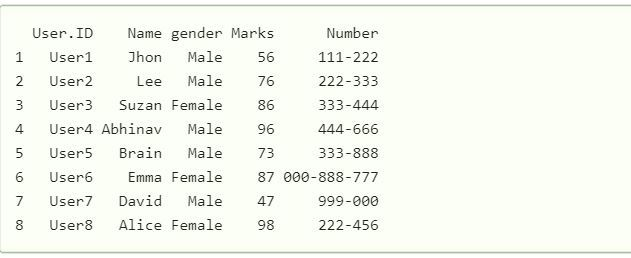Here in the above example, we have 5 vectors, Name is a character vector of length 8, User.ID is a numeric vector and all the above-mentioned vector, and using them we have created a data frame that has column length 8 and rows length 5.

Once a data frame is created we can apply various data frame operations.

#### Get Structure of a data frame:

str() function is used to get the structure of a data frame.

Example:

 `# R program to illustrate ` `# data frame from vector ` `  `  `User.ID <- ``sprintf``(``"User % d"``, 1:8)  ` `Name <- ``c``(``"Jhon"``, ``"Lee"``, ``"Suzan"``, ``"Abhinav"``, ` `          ``"Brain"``, ``"Emma"``, ``"David"``, ``"Alice"``) ` `  `  `gender <- ``c``(``"Male"``, ``"Male"``, ``"Female"``, ``"Male"``, ` `            ``"Male"``, ``"Female"``, ``"Male"``, ``"Female"``) ` `  `  `Marks <- ``c``(56, 76, 86, 96, 73, 87, 47, 98) ` `   `  `Number <- ``c``(``'111-222'``, ``'222-333'``, ``'333-444'``, ``'444-666'``, ` `            ``'333-888'``, ``'000-888-777'``, ``'999-000'``, ``'222-456'``) ` `   `  `class.df<- ``data.frame``(User.ID, Name,  ` `                      ``gender, Marks, Number) ` `str``(class.df) `

Output: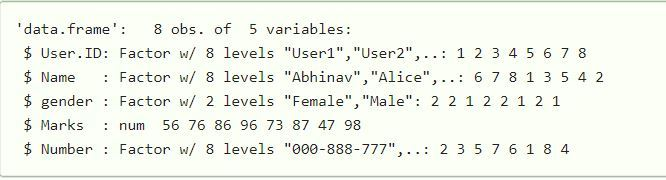Here in the above code using the `str()` function we have seen the structure of the data frame.

#### Extract Data from Data Frame

In R we can access a specific column from a data frame using the name of the column.
Example:

 `# R program to illustrate ` `# data frame from vector ` `  `  `User.ID <- ``sprintf``(``"User % d"``, 1:8)  ` `Name <- ``c``(``"Jhon"``, ``"Lee"``, ``"Suzan"``, ``"Abhinav"``, ` `          ``"Brain"``, ``"Emma"``, ``"David"``, ``"Alice"``) ` `  `  `gender <- ``c``(``"Male"``, ``"Male"``, ``"Female"``, ``"Male"``, ` `            ``"Male"``, ``"Female"``, ``"Male"``, ``"Female"``) ` `  `  `Marks <- ``c``(56, 76, 86, 96, 73, 87, 47, 98) ` `   `  `Number <- ``c``(``'111-222'``, ``'222-333'``, ``'333-444'``, ``'444-666'``, ` `            ``'333-888'``, ``'000-888-777'``, ``'999-000'``, ``'222-456'``) ` `   `  `class.df<- ``data.frame``(User.ID, Name,  ` `                      ``gender, Marks, Number) ` `extract<- ``data.frame``(class.df\$Name, ` `                     ``class.df\$gender) ` `print``(extract) `

Output: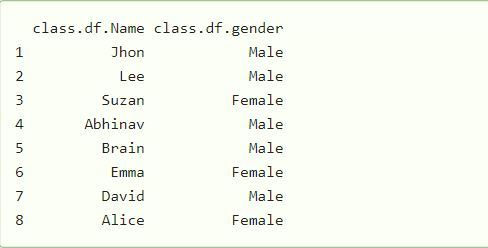#### Adding Rows and columns to data frame

To add a column we just have to add a new column vector in the data frame.
Let us take the above example to illustrate how to add a column.
Example:

 `# R program to illustrate ` `# data frame from vector ` `  `  `User.ID <- ``sprintf``(``"User % d"``, 1:8)  ` `Name <- ``c``(``"Jhon"``, ``"Lee"``, ``"Suzan"``, ``"Abhinav"``, ` `          ``"Brain"``, ``"Emma"``, ``"David"``, ``"Alice"``) ` `  `  `gender <- ``c``(``"Male"``, ``"Male"``, ``"Female"``, ``"Male"``, ` `            ``"Male"``, ``"Female"``, ``"Male"``, ``"Female"``) ` `  `  `Marks <- ``c``(56, 76, 86, 96, 73, 87, 47, 98) ` `   `  `Number <- ``c``(``'111-222'``, ``'222-333'``, ``'333-444'``, ``'444-666'``, ` `            ``'333-888'``, ``'000-888-777'``, ``'999-000'``, ``'222-456'``) ` `   `  `class.df<- ``data.frame``(User.ID, Name,  ` `                      ``gender, Marks, Number) ` ` `  `class.df\$New.column<- ``sprintf``(``"new.data % d"``, 1:8) ` `modified.dataframe <- class.df ` `print``(modified.dataframe) `

Output: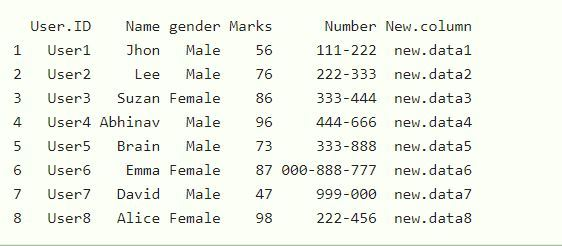Here in the above example, using the above data frame we have added a new column to the data frame, with data elements new.data.

To add a row in the data frame we have to take another data frame and we have to bind both of them using the `rbind()` function.

Let us take the above example to illustrate how to add a new row to the data frame.
Example:

 `# R program to illustrate ` `# data frame from vector ` `  `  `User.ID <- ``sprintf``(``"User % d"``, 1:8)  ` `Name <- ``c``(``"Jhon"``, ``"Lee"``, ``"Suzan"``, ``"Abhinav"``, ` `          ``"Brain"``, ``"Emma"``, ``"David"``, ``"Alice"``) ` `  `  `gender <- ``c``(``"Male"``, ``"Male"``, ``"Female"``, ``"Male"``, ` `            ``"Male"``, ``"Female"``, ``"Male"``, ``"Female"``) ` `  `  `Marks <- ``c``(56, 76, 86, 96, 73, 87, 47, 98) ` `   `  `Number <- ``c``(``'111-222'``, ``'222-333'``, ``'333-444'``, ``'444-666'``, ` `            ``'333-888'``, ``'000-888-777'``, ``'999-000'``, ``'222-456'``) ` `   `  `class.df<- ``data.frame``(User.ID, Name,  ` `                      ``gender, Marks, Number) ` ` `  `# create another data frame ` `User.ID <- ``sprintf``(``"User % d"``, 9:12) ` ` `  `Name <- ``sprintf``(``"new.data % d"``, 9:12) ` ` `  `gender <- ``c``(``"Male"``, ``"Male"``, ``"Female"``) ` ` `  `Marks <- ``c``( 87, 47, 98) ` `  `  `Number <- ``sprintf``(``"new.number % d"``, 9:12) ` `new.data<- ``data.frame``(User.ID, Name, gender, Marks, Number) ` ` `  `final.data <- ``rbind``(class.df, new.data) ` ` `  `print``(final.data) `

Output: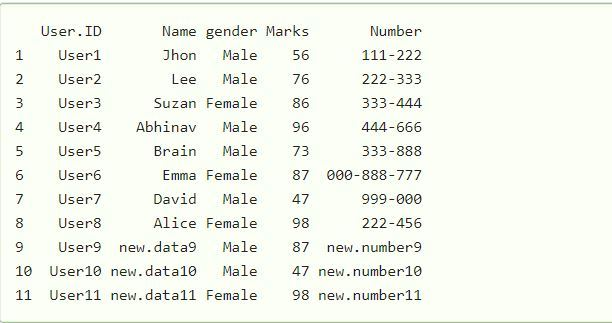Here in the above code we have created a new data frame “new.data” with 3 more rows and then bind both data frame together result in the addition of rows with “new.data”.

My Personal Notes arrow_drop_up